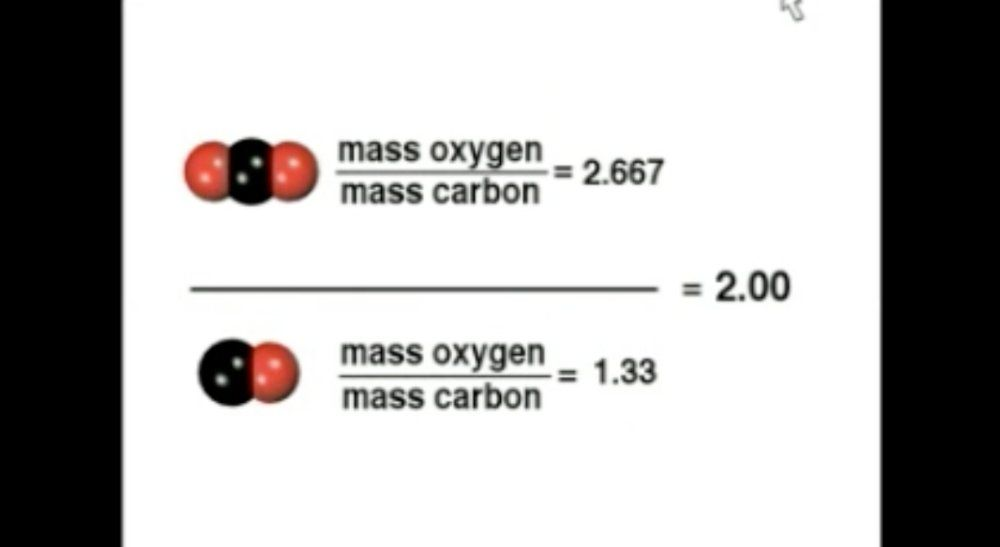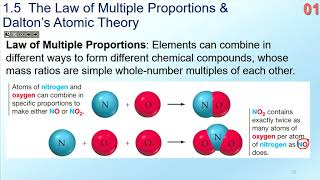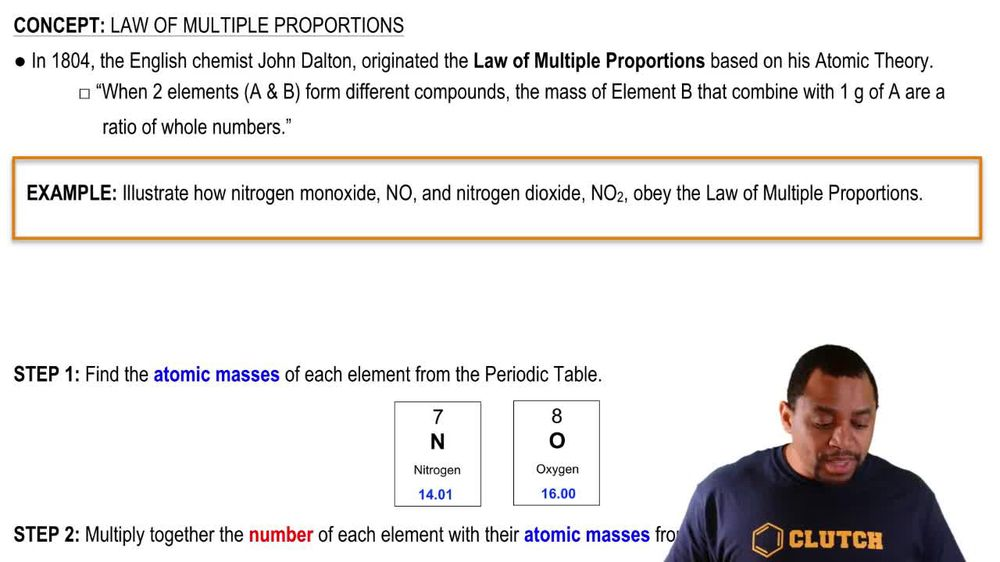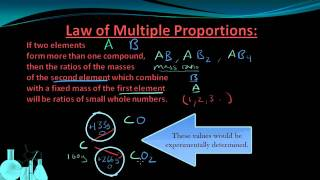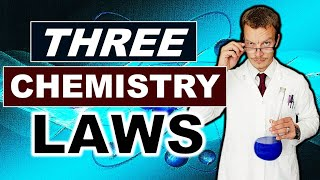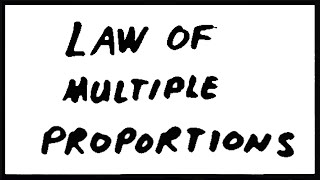Start typing, then use the up and down arrows to select an option from the list.
1. 2. Atoms & Elements2. Law of Multiple Proportions
Problem

# In a series of experiments, a chemist prepared three different compounds that contain only iodine and fluorine and determined the mass of each element in each compound: Compound Mass of Iodine (g) Mass of Fluorine (g) 1 4.75 3.56 2 7.64 3.43 3 9.41 9.86 (b) How do the numbers in part (a) support the atomic theory?

Relevant Solution5m
Play a video:
Hi everyone here we have a question telling us that a student in a chemistry lab prepared three different compounds containing only sulfur and oxygen. She then calculated the mass of each element in each of the compounds. Compound one has a mass of sulfur in grams of 3.334 and a massive oxygen and grams of 1.6 666 compound two has a massive sulfur and grams of 2.500 and a massive oxygen grams of 2.500, compound three has a mass of sulfur and grams of 2. and a massive oxygen grams of 3.0. We are told that if the mass of oxygen in each compound per unit mass of sulfur is determined and entered, your relationships are obtained. Do the obtained numbers support the atomic theory. So we first need to calculate the oxygen to sulfur mass ratio and we're gonna do that by dividing the mass of oxygen by the mass of sulfur. So for compound one we have 1.666g of oxygen, Divided by 3. g of sulfur, and that equals 0. g of oxygen for every one g of sulfur. Next We have 2.500 g of oxygen divided by 2.500 g of sulfur, and that equals 1.0 g of oxygen. For every one g of sulfur. Lastly we have 3.000 g of oxygen Divided by 2.000 g of sulfur. And that equals 1. g of oxygen for every one g of sulfur. And now we want to find our integral relationships. So to do that, we're going to divide these numbers by the smallest number that we got, which was 0.4997. And then we're going to round all of the numbers. So for compound one That would be 0.4997, Divided by 0.4997. And that equals 1.000 or one. For compound to that would be 1.000 divided by 0.4997. And that equals 2.001. Or we can round that to two And then for compound three we have 1. Divided by 0. And that equals 3.002. Or we could round that to three. So as we can see these are all really close to whole numbers and according to the atomic theory, atoms are indivisible. And this supports that theory because the integral ratios indicate that the oxygen atoms that combine with sulfur atoms are indivisible because they are in whole numbers. So our final answer is yes. This does support the atomic theory. Thank you for watching. Bye